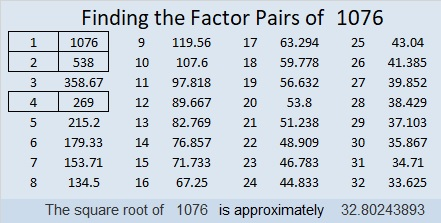# 1076 and Level 4

If you roll a pair of dice, you are taking a chance that the roll might not be favorable for you. Don’t take your chances when solving this puzzle. It can be solved completely by relying on logic. It won’t require any luck, but “Good luck!” anyway.Print the puzzles or type the solution in this excel file: 10-factors-1073-1079

Here is some information about the number 1076:

• 1076 is a composite number.
• Prime factorization: 1076 = 2 × 2 × 269, which can be written 1076 = 2² × 269
• The exponents in the prime factorization are 2 and 1. Adding one to each and multiplying we get (2 + 1)(1 + 1) = 3 × 2  = 6. Therefore 1076 has exactly 6 factors.
• Factors of 1076: 1, 2, 4, 269, 538, 1076
• Factor pairs: 1076 = 1 × 1076, 2 × 538, or 4 × 269
• Taking the factor pair with the largest square number factor, we get √1076 = (√4)(√269) = 2√269 ≈ 32.802441076 is the hypotenuse of a Pythagorean triple:
276-1040-1076 which is 4 times (69-260-269)

1076 is a palindrome in a couple of bases:
It’s 434 in BASE 16 because 4(16²) + 3(16) +4(1) = 1076, and
it’s 1I1 in BASE 25 (I is 18 base 10) because 25² + 18(25) + 1 = 1076

This site uses Akismet to reduce spam. Learn how your comment data is processed.